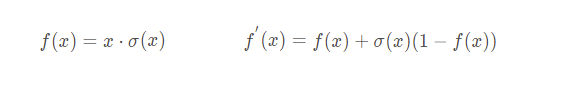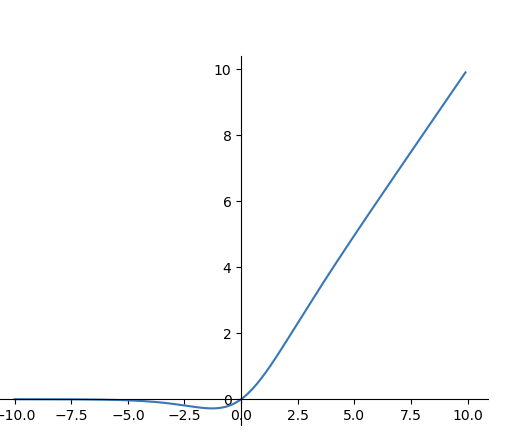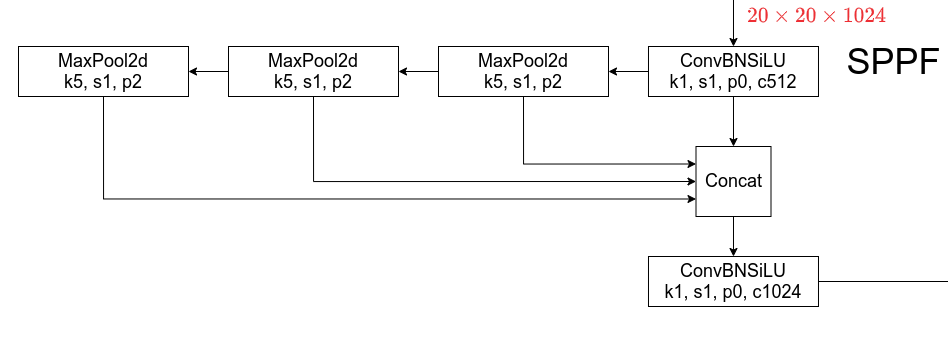Yolov5 已经更新到v6.1版本了，与之前的版本有了不少区别，网络结构有了进一步优化。来整理一下。## 删除Focus层## 激活函数改用SiLU## SPPF

yolov5之前的版本的Neck结构采用了SPP模块。class SPP(nn.Module):
# Spatial Pyramid Pooling (SPP) layer https://arxiv.org/abs/1406.4729
def __init__(self, c1, c2, k=(5, 9, 13)):
super().__init__()
c_ = c1 // 2  # hidden channels
self.cv1 = Conv(c1, c_, 1, 1)
self.cv2 = Conv(c_ * (len(k) + 1), c2, 1, 1)
self.m = nn.ModuleList([nn.MaxPool2d(kernel_size=x, stride=1, padding=x // 2) for x in k])

def forward(self, x):
x = self.cv1(x)
with warnings.catch_warnings():
warnings.simplefilter('ignore')  # suppress torch 1.9.0 max_pool2d() warning
return self.cv2(torch.cat([x] + [m(x) for m in self.m], 1))

class SPPF(nn.Module):
# Spatial Pyramid Pooling - Fast (SPPF) layer for YOLOv5 by Glenn Jocher
def __init__(self, c1, c2, k=5):  # equivalent to SPP(k=(5, 9, 13))
super().__init__()
c_ = c1 // 2  # hidden channels
self.cv1 = Conv(c1, c_, 1, 1)
self.cv2 = Conv(c_ * 4, c2, 1, 1)
self.m = nn.MaxPool2d(kernel_size=k, stride=1, padding=k // 2)

def forward(self, x):
x = self.cv1(x)
with warnings.catch_warnings():
warnings.simplefilter('ignore')  # suppress torch 1.9.0 max_pool2d() warning
y1 = self.m(x)
y2 = self.m(y1)
return self.cv2(torch.cat([x, y1, y2, self.m(y2)], 1))


## 网络输出

yolov5 经过5次下采样，最大会放缩32倍，原图的长宽都要求是32的倍数。v6.0版本将图片的输入默认改为640，也就是说输出的结果是20*20*(5+n)40*40*(5+n)80*80*(5+n)。n为分类的总数。

## 参考

1. https://blog.csdn.net/qq_37541097/article/details/123594351